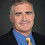## Thursday, March 16, 2017

### NP in ZPP implies PH in ZPP

If NP is in ZPP is the entire polynomial-time hierarchy in ZPP? I saw this result used in an old TCS Stackexchange post but I couldn't find a proof (comment if you know a reference). The proof that NP in BPP implies PH in BPP is harder than it looks and NP in BQP implies PH is in BQP is still open as far as I know.

I found a simple proof that NP in ZPP implies PH in ZPP and then an even simpler one.

Assume NP in ZPP. This implies NP in BPP so PH is also in BPP. So we need only show BPP in ZPP.

BPP is in ZPPNP follows directly by Lautemann's proof that BPP is in Σ2P or by the fact that BPP is in MA is in S2P is in ZPPNP. By assumption, BPP in ZPPNP implies BPP in ZPPZPP = ZPP.

And this is even simpler.

ZPP = RP∩co-RP in NP∩co-NP. Σ2P = NPNP in NPZPP (by assumption) in NPNP∩co-NP = NP in ZPP. You can get the higher levels of the hierarchy by an easy induction.

1.As can be seen from your second argument, you practically use that ZPP is in co-NP and thus NP is in co-NP, which implies (as you have sketched) that NP=PH.

2.You can also say that NP is in ZPP implies that NP is in BPP (since ZPP is in BPP), so by Karp-Lipton theorem PH=Σ2, and so
PH=Σ2=NP^NP which, by assumption is in ZPP^ZPP=ZPP.

1.NP in ZPP implies NP^NP in NP^ZPP but not directly that NP^ZPP in ZPP^ZPP.

3.In proposition 1.3 in https://www.semanticscholar.org/paper/The-Satanic-Notations%3A-Counting-Classes-beyond-%23p-1-Hemaspaandra/c01bebe8da499eb6a585c36cde9a71a406db41e6 it is shown

#.NP=#.P^NP iff NP=UP^NP iff NP=coNP.

NP in ZPP implies NP=coNP and so ZPP=NP=UP^NP=UP^ZPP holds and so is ZPP low for UP providing ZPP=UP?

Thank you.

4.In proposition 1.3 in https://dl.acm.org/doi/abs/10.1145/203610.203611 it is stated #.NP=#.P^NP iff NP=UP^NP iff NP=coNP.

NP in ZPP implies NP=coNP and so ZPP=NP=UP^NP=UP^ZPP holds and so is ZPP low for UP providing ZPP=UP?

5.Full question.

Explicitly I want to identify relation between UP and ZPP since we do not even know ZPP is in SPP although we believe it to be true.
In proposition 1.3 in https://dl.acm.org/doi/abs/10.1145/203610.203611 it is stated #.NP=#.P^NP iff NP=UP^NP iff NP=coNP.
Theorem 7.2 in On counting and approximation (springer.com) states span-P is approximable in BPP^NP.
NP in ZPP implies span-P is approximable in ZPP.

1. span-P is in #(P^NP) and soI wonder if NP=ZPP would we get span-P in #P and so by theorem 4.9 UP=NP=ZPP?

Ordinarily is ZPP low for NP? So we get:

2. NP in ZPP implies NP=coNP and so ZPP=NP=UP^NP=UP^ZPP holds and so is ZPP low for UP providing NP=ZPP=UP?
So could NP=ZPP have any implications to UP and ZPP.

Assume further UP=coUP and so UPH collapses to UP. We already assumed NP=ZPP holds. So UP is in ZPP and in NP.

3. At least would it say something about the lowness UP making ZPP^UP in UP?

4. coUP=UP in EP in NP=ZPP=coNP holds under assumptions. EP is known to be in SPP. Is EP known to be in UPH under the assumptions?

6.Can someone explain why ZPP^ZPP = ZPP?

1.ZPP means always correct in expected poly time. So a ZPP^ZPP machine just simulates the oracle with its queries. Since the expectation of a sum is the sum of the expectations, the poly # of queries can be simulated in an expected polynomial number of steps.

7.Can you elaborate why \Sigma_2^{P} \subseteq ZPP^{NP}?

1.Probably not because that would imply Simga_2 in Pi_2 and the polynomial-time hierarchy collapses.